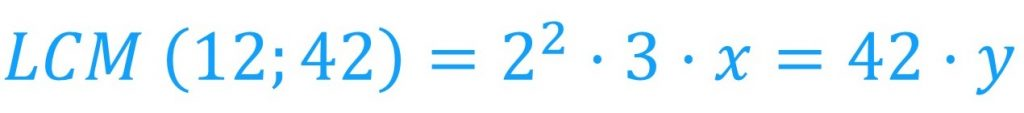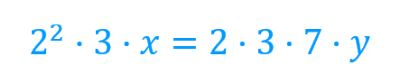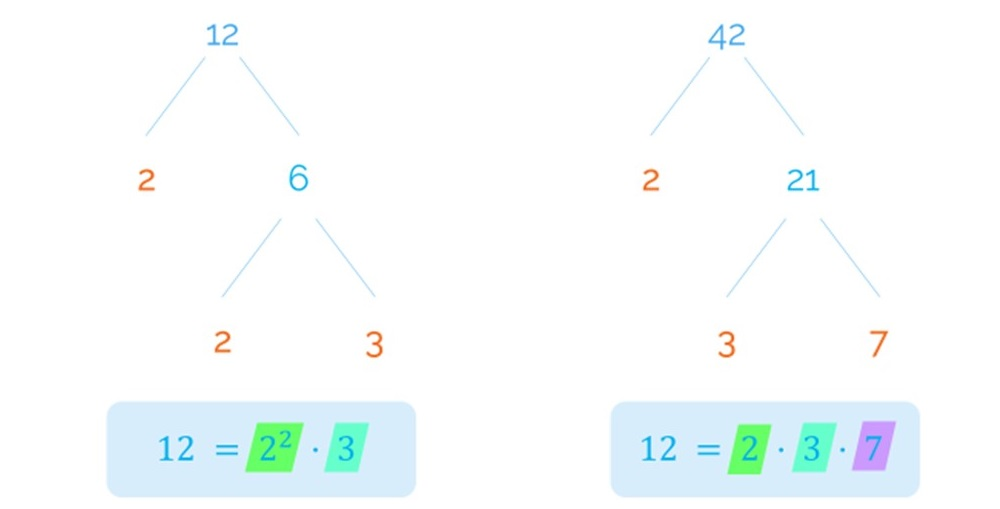Smartick is a fun way to learn math!Jul23

# Explanation of the Formula to Calculate the Least Common Multiple

I am sure this sounds familiar to many of you: To calculate the least common multiple (LCM), we have to choose between the common and uncommon factors that have the highest exponent.

But what does it mean? Why do we have to choose from all factors…and out of the common factors, why the ones with the highest exponent? Let’s find out!

The least common multiple is the lowest common multiple of two or more numbers, as we explained in the post: How to Calculate LCM?

Alright, now let’s analyze another very important element of our research: the factorization into prime numbers. All numbers have their own prime factorization—it is just like the ID of numbers.

For example, the “ID” of 12 is 2^2·3, and the “ID” of 42 is 2·3·7.

Now, we are going to use these two numbers as an example to discover the reasons behind the sentence with which we started the post.

When we calculate the least common multiple of two numbers (in this case), we are looking for the lowest multiple that is, at the same time, a multiple of both numbers.

In this case, it is the least common multiple of 12 and 42 [LCM (12; 42)] which is equal to 12 multiplied by x or 42 multiplied by y:As we have said that the factorization of 12 is 2^2·3, we can say that:As we have said that the factorization of 42 is 2·3·7, we can say that:Great. Now we are going to take a closer look at this particular part of the expression:On both sides we need the same prime factors, because we are looking for the same number that we are going to write according to its prime factorization.

We see that the factorization of this number will have 3 in both of the expression’s terms, and 2 squared that is only located in the term on the left. Therefore, we know that y will be 2.If we look closely at the term on the right, we can see that “that number”, the least common multiple of 12 and 42, would also have 7 as a factor that is not in the term on the left. This way, we know that x is 7, and we get:If we group together the powers of the common factors, we will get the same expression on both sides:This means that the least common multiple of 12 and 42 is 84:These factors are all of those that 12 and 42 do not have in common (in this case it is 7) and of the common factors, it is the one that has the highest exponent. The common factors are 2 and 3; 3 has an exponent of 1 in both cases, but between the “twos” we will choose the one with the highest exponent: 2 squared.What do you think? Did you already know how to do this?

If you would like to keep learning about similar concepts, go to Smartick and try it for free.